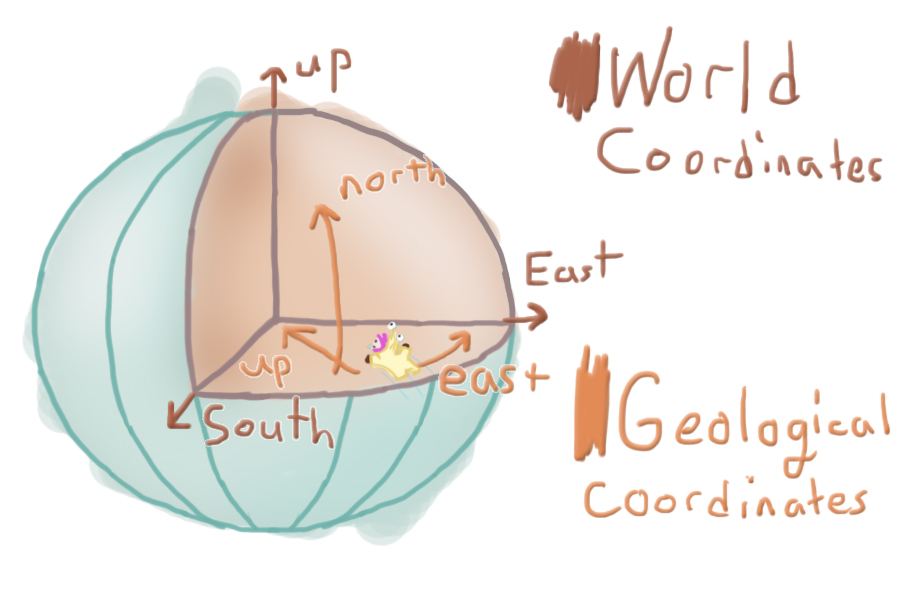Problems Programming In An Inverted World: Directionality

How we've programmed directionality and orientation in an inverted planet.

Posted by on

Here at PolyKnight Games, we’re making InnerSpace, which takes place in inverted planetoids. This is really cool, but it brings up a lot of technical issues. There are things we can’t do the typical way. We share plenty of technical issues that are faced by games that use regular planetoids, but with some added “fun” on the development side. That being said, I’ll cover the core of the issue.

We face two base issues:

1. Which ways are up, north, and east?
2. What are my current coordinates?

The first thing to define is up, north, and east, based on your location in the sphere. In defining this we need to create arrows that point in the direction you must travel to reach the center of the sphere (up), to reach the north pole (north), and to reach what we’ll call the east-pole (east). The east-pole is the eastern most point of the planet, just like the north-pole is the northern most point. This then defines a “local” coordinate system, which tells us which way is up, north, and south, see the picture below.

WARNING: Programmer ArtNext up we want to utilize a different coordinate system, which describes the player’s location inside the sphere. The traditional coordinate system is called Cartesian coordinates and is organized in 3d-space with three values: (x, y, z). This is a fine coordinate system, as the y value normally describes your distance from the ground and the x and z coordinates describe your location in space, but this isn’t fitting for a sphere. Y does not define distance from the ground anymore and x nor z describe distance traveled. Geographical coordinates are defined by angle from the equator and angle traversed around the equator. It utilizes longitude and latitude, which replace x and z for distance traveled, and height, which replaces y for distance off the ground. Calculating this isn’t as useful in a technical sense, although it’s very useful to the player. This coordinate makes traversing the planet: #1 feel very official, and #2 Is more organized. It’s easier to read and is less information than (x, y, z) coordinates. The height, however, is useful. We exclusively use this calculation for plotting maps, as the player takes the role of a cartographer.

If you’re interested in this from a programming perspective, you’ll want to make sure you’re fresh on your vector operations and your trigonometry. I use a lot of Unity’s operations for example sake, but you’ll likely want to write out these equations yourself (I won’t explain the difference between vector operations / calling Vector3.Angle(a, b) and using a Asin(theta)/Dot product with proper vector projections (which accounts for planetary rotation)).

## What this affects

• Direction of gravity (which way is down/up?)
• Used to orient AI, so birds don’t fly upside down
• Physics objects, so they don’t fly sideways
• Curve fitting/modeling, so the plane dives to the closest water
• Height into atmosphere or distance from water surface
• Height based effects, so the water russles
• Physics object gravity strength, if we want gravity to get stronger
• AI pathfinding, so something can fly along the water’s surface
• Player map & coordinates, so we we know where we are at and can mark key locations logically
• Pretty much everything## Orientation

We will solve based on object location relevant to the current planet. As you can see in the image above, green denotes up, blue defines north, and red defines east. The thinner lines attached to each of the spheres denote the recalculated directionality.

The east and north are calculated as tangents of the arc, meaning they are representative of the sphere’s curvature at that exact point as a straight line. They do not point to the pole, but instead the direction you would need to travel to reach the north or eastern poles, while maintaining your current height.

Here’s some pseudo-code:

• Define up based on relative position to planet.
• objectUp = (objectPosition – planetPosition) * -1
• Use cross products to derive east and north
• objectEast= Cross(worldUp, objectUp)
• objectNorth = Cross(objectUp, objectEast)

## Coordinates

For coordinates, we will convert our typical Cartesian coordinates (x, y, z) to geographic coordinates (longitude, latitude, and height). For usual geographical coordinates, height (ht) is defined by distance off the surface (further from center). For InnerSpace we’re backwards: height is defined by distance from the center (closer to the center). Height becomes negative as you go further into the water, or past the surface (further from the center). I calculate longitude and latitude the same as a standard (spherical) planet, though.

Here’s some pseudo-code:

• Project the object’s relative position onto the planet’s xz-plane
• vMod = pos – planetPos
• vMod.y = 0
• Calculate longitude as the angle between the planet’s forward and your projected vector.
• l planet.forward)
• if (relPos.x < 0) longitude *= -1
• turns the value negative if it’s on the dark-side of the planet
• Calculate latitude as the angle between the projected vector and the actual relative vector.
• latitude = Angle(vMod, relPos)
• if (relPos.y < 0) latitude*= -1f
• turns the value negative if it’s on the dark-side of the planet
• Calculate the height based on the relative position of the object and the planet’s radius. Invert the value.
• height = (relPos – planetRadius) * -1